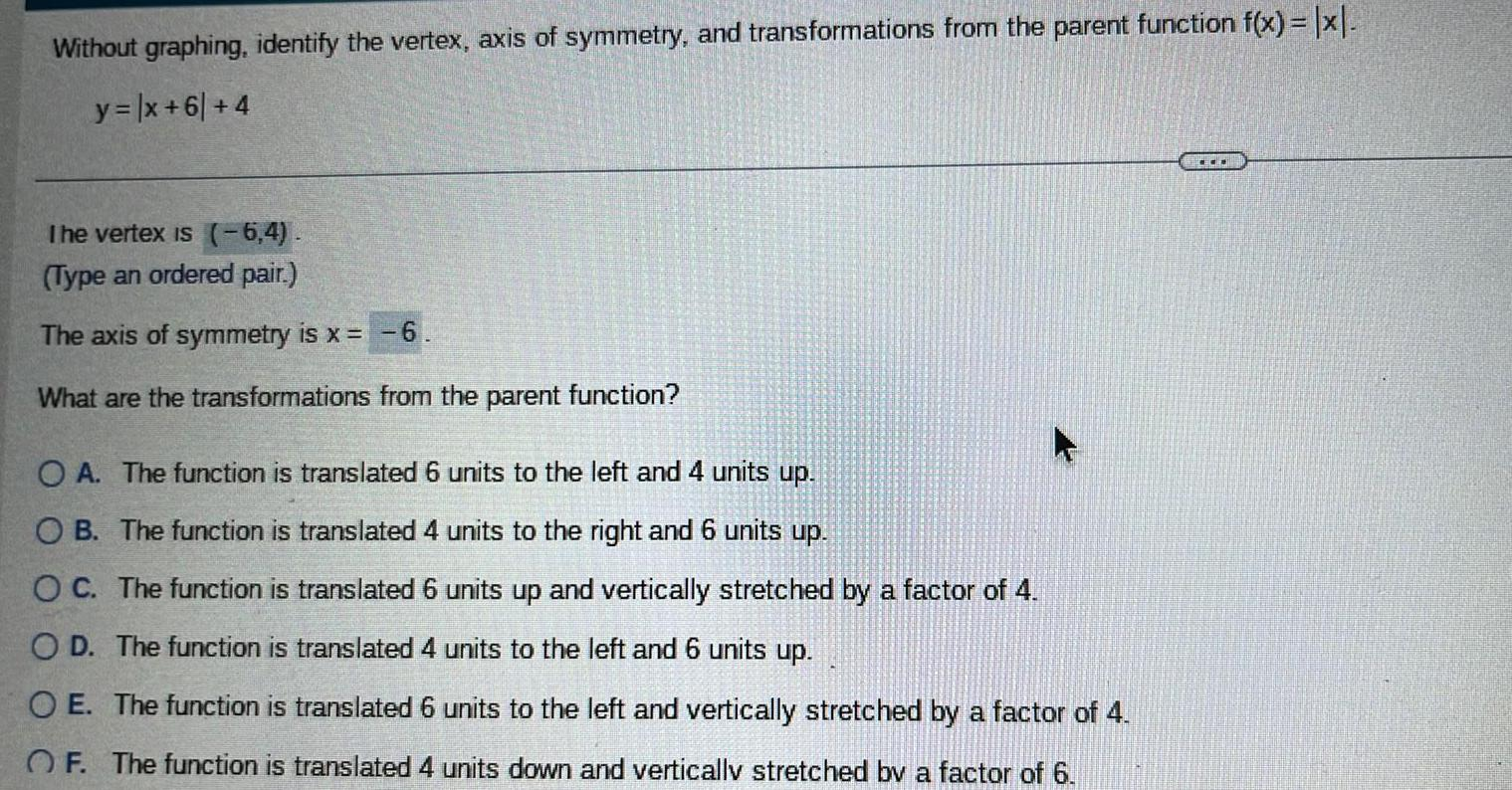Question:

# Without graphing identify the vertex axis of symmetry and

Last updated: 9/14/2023Without graphing identify the vertex axis of symmetry and transformations from the parent function f x x y x 61 4 The vertex is 6 4 Type an ordered pair The axis of symmetry is x 6 What are the transformations from the parent function O A The function is translated 6 units to the left and 4 units up OB The function is translated 4 units to the right and 6 units up OC The function is translated 6 units up and vertically stretched by a factor of 4 OD The function is translated 4 units to the left and 6 units up OE The function is translated 6 units to the left and vertically stretched by a factor of 4 OF The function is translated 4 units down and vertically stretched by a factor of 6